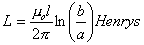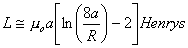# Inductance Formulas

The formulas on this page allow one to calculate the inductance for certain given geometries.  More complex problems typically require one to model the geometry and solve using Finite Element Analysis.  The viewer should note that the permeability has been set to that of free space for these formulas since that is typically the most common scenario in calculating the inductance of a transmission line, cable, etc.

Other inductance formulas can also be found in the following references:

Pulsed Power Toolbox Formulas for the Calculation of Common Inductances

Inductance Calculations Working Formulas and Tables, Frederick W. Grover, Dover Publications, Inc., New York, New York, 1946.

Radio Engineers Handbook, Frederick E. Terman, McGraw-Hill Book Company, New York, New York, 1943.

“Formulas for the Skin Effect”, Harold A. Wheeler, Proceedings of the I.R.E., September, 1942, pp. 412-424.

2 Parallel Plane PlatesDiagram for Parallel Plate Inductance CalculationEquation for Parallel Plate Inductance Calculation m0 is the permeability of free space (H/m) d is the distance between the plates (m) l is the length of the electrodes (m) w is the width of the electrodes (m)

2 Coaxial CylindersDiagram for Coaxial Cylinder Inductance CalculationEquation for Coaxial Cylinder Inductance Calculation m0 is the permeability of free space (H/m) a is the radius of the inner cylinder b is the radius of the outer cylinder l is the length of the cylinders (m)Diagram for Wire Loop Inductance CalculationEquation for Wire Loop Inductance Calculation m0 is the permeability of free space (H/m) a is the radius of the wire loop (m) R is the radius of the wire (m)